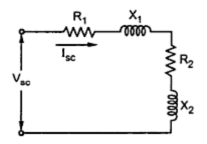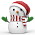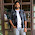### Conducts Tests on Single Phase Induction Motor

Similar to a three phase induction motor, the various tests can be performed on single phase induction motor. The results of these tests can be used to obtain the equivalent circuit parameters of a single phase induction motor. The tests usually conducted are :
1. No load test or open circuit test
2. Blocked rotor test or short circuit test

The test is conducted by rotating the motor without load. The input current, voltage and power are measured by connecting the ammeter, voltmeter and wattmeter in the circuit. These readings are denoted as Vo , Io and Wo .
Now                 Wo = Vo Io cosΦ

The motor speed on no load is almost equal to its synchronous speed hence for practical purposes, the slip can be assumed zero. Hence r2/s becomes ∞ and acts as open circuit in the equivalent circuit. Hence for forward rotor circuit, the branch r2/s + j x2 gets eliminated.
While for a backward rotor circuit, the term r2/(2 - s) tends to r2/2. Thus xo is much higher then the impedance r2/2 + j x2. Hence it can be assumed that no current can flow through and that branch can be eliminated.
So circuit reduces to as shown in the Fig.1.Fig. 1

Now the voltage across xo is VAB
But                    VAB = Io xo
...                       xo = VAB /Io
But                   xo = Xo /2

Thus magnetising reactance Xo can be determined.
The no load power Wo is nothing but the rotational losses.

2. Blocked Rotor Test
In balanced rotor test, the rotor is held fixed so that it will not rotate. A reduced voltage is applied to limit the short circuit current. This voltage is adjusted with the help of autotransformer so that the rated current flows through main winding. The input voltage, current and power are measured by connecting voltmeter, ammeter and wattmeter respectively. These readings are denoted as Vsc , Isc and Wsc.
Now as rotor is blocked, the slip s = 1 hence the magnetising reactance xo is much higher than the rotor impedance and hence it can be neglected as connected in parallel with the rotor. Thus the equivalent circuit for blocked rotor test is as shown in the Fig.2.Fig. 2

Wsc = Vsc Isc cos Φsc
cos Φsc =Wsc /Vsc Isc
= blocked rotor power factor
Now               Zeq = Vsc/ Isc
Req = Wsc /( Isc)2
But                  Req = R1 + R2
...                     R2 = Req - R1
= rotor resistance referred to stator
Xeq =√(Zeq 2 - Req 2)
X1 =  Xwe get,

The stator resistance is measured by voltmeter-ammeter method, by disconnecting the auxiliary winding and capacitors present if any. Due to skin effect, the a.c. resistance is 1.2 to 1.5 times more than the d.c. resistance.
Key point : Thus with two tests, all the parameters of single phase induction motor can be obtained.

1.Thanks....Good Explanation.....

2.Thnqqq.... Explained clearly

3.Thnqqq.... Explained clearly

4.Thanks for the explanation

5.6.After reading here no need to study book.Thanks good explanation.